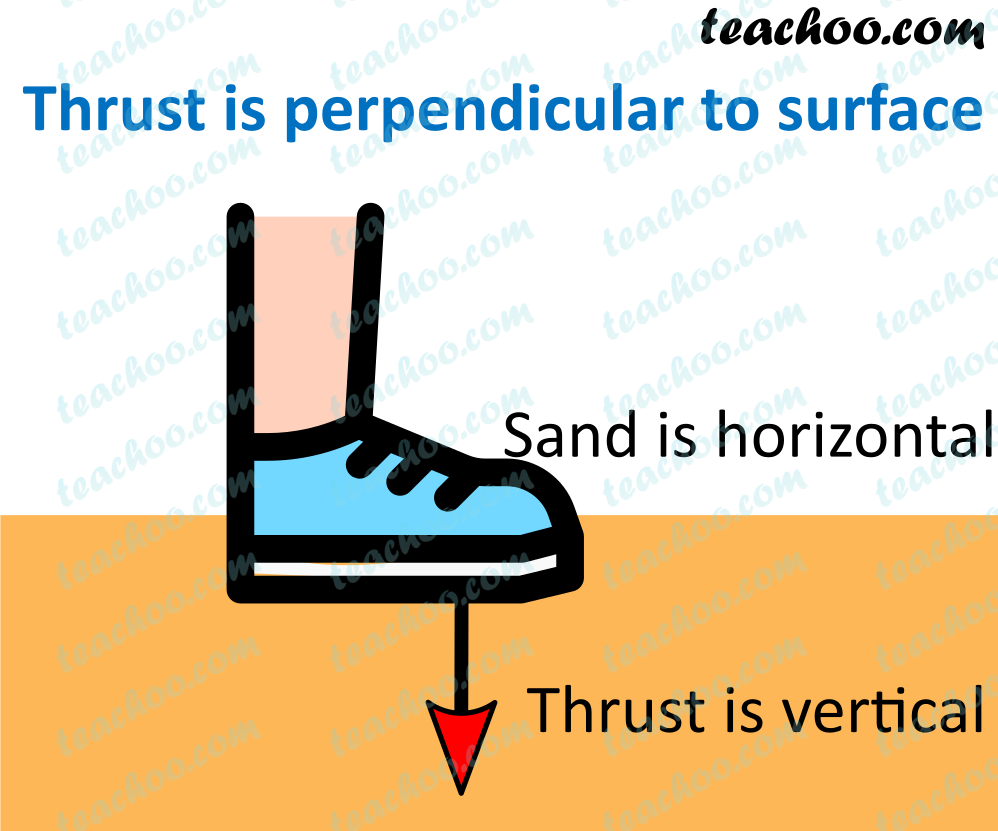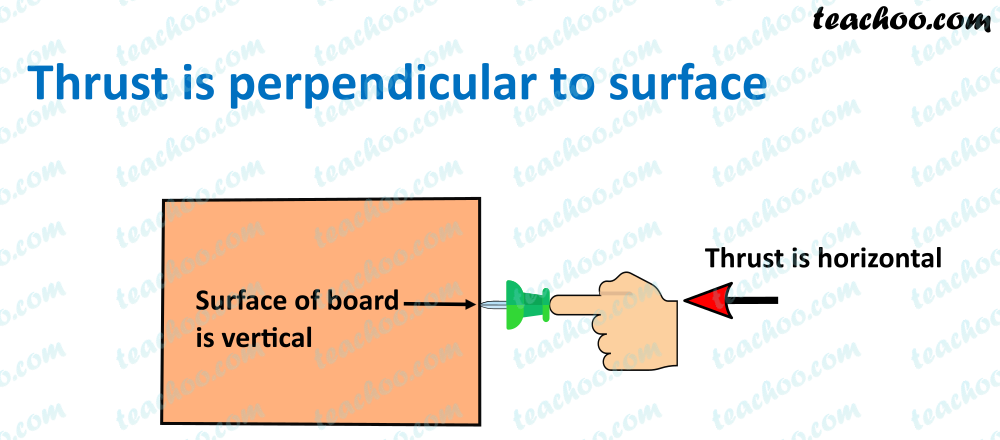Concepts

Class 9
Chapter 10 Class 9 - Gravitation

Force acting on an object perpendicular to surface is called thrust

It is net force acting in a particular direction

It is always perpendicular to surface on which force applied

Example

When we stand on loose sand,our body is exerting force on sand by our feet

Surface of Sand is horizontal but our feet is standing vertically on it

It can be seen that our feet are applying force perpendicular to surface area

This force is called thrust

##googletag.cmd.push(function() { googletag.display('div-gpt-ad-1669298377854-0'); });

Example 2

When we fix a poster to bulletin board

We fix a pin to it

It can be seen that bulletin board is vertically placed

we apply force in perpendicular direction by pressing pin to it

This force is called thrust

##(adsbygoogle = window.adsbygoogle || []).push({});

What is formula of thrust?

Since thrust is also a force

Its formula is same as force

Thrust = Mass × Acceleration = m × a = m × g

Since it is a force,its SI Unit is also Newtons

Force = Mass × Acceleration

F = m × a

Acceleration due to gravity is denoted by g,So formula becomes

F = m × g

Since thrust is also a force,we can also write that

Thrust = f = m × g

Learn in your speed, with individual attention - Teachoo Maths 1-on-1 Class